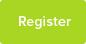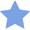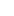# Tutor Hunt Questions

1. If you write numbers as words, what is the first number to have an ‘a’ in it?

2. What is the next palindromic number after 72927? (A palindromic number reads the same forward and backward)?

3. If we add 12 thousand, 12 hundred and 12 what is the largest digit in the answer?

3 years ago

Please enter your response to the question below. The student will get a notification as soon your response has been approved by our moderation team.

####Accepted Answer

1. So as none of the numbers 1 through 10 contain an a, we look at 11, 12 and the teens no a`s there either. It all gets pretty repetitive after that, so we should focus on the multiples of 10 (20,30 etc).all the way up to 99. Still no a`s. 100? No a`s.

101? Now the word `and` is used so maybe this counts, but and isn`t a number so the next one after this will be 1000.

2. normally to count up we increase the value of the unit, but as this is a palindrome the smallest increment will be the middle digit. In this case a 9. Increases the 9 will knock on to the thousands column so the next palindromic number will begin 73___. Therefore end ___37. The `next` palindromic number will have the lowest possible value in the hundreds column.

3. 12 thousand is written as 12 with three zeros so the 2 is in the thousands column. Adding 12 hundred means another 1 is added to the thousands column and the 2 will be in the hundreds column. The final number to add is 12. This doesn`t increase the value of any digits in higher place values.

Therfore the highest value digit will be the sum of the thousands column digits.
Answered by Danielle | 3 years ago
1. one hundred and one.
2. 73037
3. the one in the place of ten thousand - or were you asking the biggest individual digit? (in which case you were thinking of the three in 13212)
Answered by Gina | 3 years ago
(1 )question answer is .... 2

(2 )question answer is .... 73037